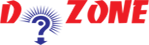﻿ How to find the largest among three numbers using python coding?## Python Program To Find the Largest Among Three Numbers

Here, in this program you will learn how to find the largest among the three numbers by using if else and display. the three numbers are entered in as num1, num2 and num3 respectively. The if, elif and else is the ladder that used to find the largest among the three numbers.

``````
Input
# Python program to find the largest number among the three input numbers

# change the values of num1, num2 and num3

# for a different result

num1 = 11

num2 = 18

num3 = 14

# uncomment following lines to take three numbers from user

#num1 = float(input("Enter first number: "))

#num2 = float(input("Enter second number: "))

#num3 = float(input("Enter third number: "))

if (num1 >= num2) and (num1 >= num3):

largest = num1

elif (num2 >= num1) and (num2 >= num3):

largest = num2

else:
largest = num3

print("The largest number between",num1,",",num2,"and",num3,"is",largest)

Output
The largest number between 11, 18 and 14 is 18.0
```
```

## Other Python Programs

PythonTraining, 258-FF , Katewa Nagar, New Sanganer Rd, Jaipur, 302019